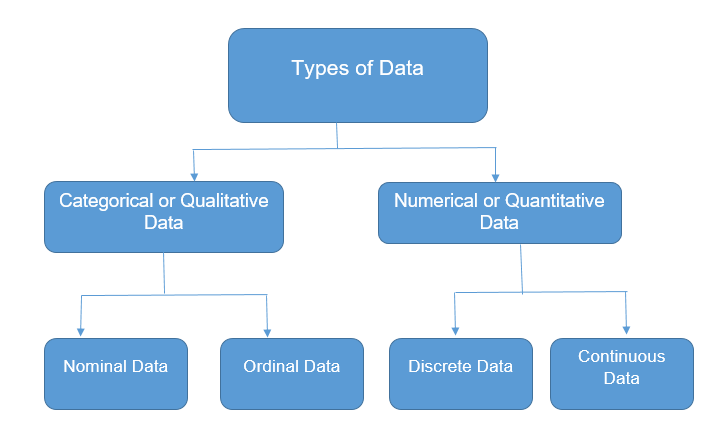# Types of Data in Statistics

In Statistics, the data are the individual pieces of factual information recorded, and it is used for the purpose of the analysis process. The two processes of data analysis are interpretation and presentation. Statistics are the result of data analysis. Data classification and data handling are an important process as it involves a multitude of tags and labels to define the data, its integrity and confidentiality. In this article, we are going to discuss the different types of data in statistics in detail.

## Types of Classification of Data in Statistics

The data in Statistics are classified as follows:Let us discuss the different types of data in Statistics herewith examples.

## Qualitative or Categorical Data

Qualitative data, also known as the categorical data, describes the data that fits into the categories. Qualitative data are not numerical. The categorical information involves categorical variables that describe the features such as a person’s gender, home town etc. Categorical measures are defined in terms of natural language specifications, but not in terms of numbers.

Sometimes categorical data can hold numerical values (quantitative value), but those values do not have mathematical sense. Examples of the categorical data are birthdate, favourite sport, school postcode. Here, the birthdate and school postcode hold the quantitative value, but it does not give numerical meaning.

### Nominal Data

Nominal data is one of the types of qualitative information which helps to label the variables without providing the numerical value. Nominal data is also called the nominal scale. It cannot be ordered and measured. But sometimes, the data can be qualitative and quantitative. Examples of nominal data are letters, symbols, words, gender etc.

The nominal data are examined using the grouping method. In this method, the data are grouped into categories, and then the frequency or the percentage of the data can be calculated. These data are visually represented using the pie charts.

### Ordinal Data

Ordinal data/variable is a type of data which follows a natural order. The significant feature of the nominal data is that the difference between the data values is not determined. This variable is mostly found in surveys, finance, economics, questionnaires, and so on.

The ordinal data is commonly represented using a bar chart. These data are investigated and interpreted through many visualisation tools. The information may be expressed using tables in which each row in the table shows the distinct category.

## Quantitative or Numerical Data

Quantitative data is also known as numerical data which represents the numerical value (i.e., how much, how often, how many). Numerical data gives information about the quantities of a specific thing. Some examples of numerical data are height, length, size, weight, and so on. The quantitative data can be classified into two different types based on the data sets. The two different classifications of numerical data are discrete data and continuous data.

### Discrete Data

Discrete data can take only discrete values. Discrete information contains only a finite number of possible values. Those values cannot be subdivided meaningfully. Here, things can be counted in the whole numbers.

Example: Number of students in the class

### Continuous Data

Continuous data is data that can be calculated. It has an infinite number of probable values that can be selected within a given specific range.

Example: Temperature range

To learn more on Statistics, visit BYJU’S – The Learning App and download the app to explore more Maths-related videos to learn with ease.

Test your knowledge on Types Of Data In Statistics